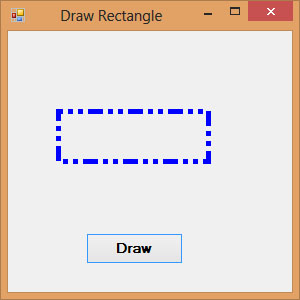# Visual Basic 2010 Lesson 22 – The DrawRectangle Method

### 22.1 Creating a Rectangle with DrawRectangle Method

There are two methods to draw a rectangle on the screen in VB2010:

#### Method 1

Use the DrawRectangle method by specifying its upper-left corner’s coordinate and it width and height. You also need to create a Graphics and a Pen object to handle the actual drawing. The syntax is:

`myGrapphics.DrawRectangle(myPen, X, Y, width, height)`

*myGraphics is the variable name of the Graphics object and myPen is the variable name of the Pen object created by you. X, Y is the coordinate of the upper left corner of the rectangle.

#### The code

```Dim myPen As Pen
myPen = New Pen(Drawing.Color.Blue, 5)
Dim myGraphics As Graphics = Me.CreateGraphics
myGraphics.DrawRectangle(myPen, 0, 0, 100, 50)
```

#### Method 2

Create a rectangle object first and then draw this rectangle using the DrawRectangle method. The syntax is as shown below:

```myGraphics.DrawRectangle(myPen,myRectangle)
```

where myRectangle is the rectangle object created by you, the user.

The code is:

```Dim myRectangle As New Rectangle
myRect.X = 10
myRect.Y = 10
myRect.Width = 100
myRect.Height = 50
```

You can also create a rectangle object using a one-line code as follows:

```Dim myRectangle As New Rectangle(X,Y,width, height)
```

and the code to draw the above rectangle is

```myGraphics.DrawRectangle(myPen, myRectangle)
```

### 22.2 Customizing Line Style of the Pen Object

The shape we draw so far are drawn with a solid line, we can actually customize the line style of the Pen object so that we have dotted line, a line consisting of dashes and more. For example, the syntax to draw a dotted line is shown below:

```myPen.DashStyle=Drawing.Drawing2D.DashStyle.Dot
```

Where the last argument Dot specifies a particular line DashStyle value, a line that makes up of dots here. The following code draws a rectangle with the red dotted line.

```Private Sub Button1_Click(ByVal sender As System.Object, ByVal e As System.EventArgs) Handles Button1.Click
Dim myPen As Pen
myPen = New Pen(Drawing.Color.Red, 5)
Dim myGraphics As Graphics = Me.CreateGraphics
myPen.DashStyle = Drawing.Drawing2D.DashStyle.Dot
myGraphics.DrawRectangle(myPen, 10, 10, 100, 50)
End Sub
```

The output image is shown below:###### Figure 22.1

If you change the DashStyle value to DashDotDot, you can draw rectangles with different border, as shown in Figure 22.2.

The possible values of the line DashStyle of the Pen are listed in the table below:

DashStyle
Value
Line Style
Dot Line consists of dots
Dash Line consists of dashes
DashDot Line consists of alternating dashes and
dots
DashDotDot Line consists of alternating dashes and
double dots
Solid Solid line
Custom Custom line style###### Figure 22.2

[Lesson 21] << [CONTENTS] >> [Lesson 23]